# Group algebra

(diff) ← Older revision | Latest revision (diff) | Newer revision → (diff)

of a groupover a fieldThe associative algebra (cf. Associative rings and algebras) overwhose elements are all possible finite sums of the type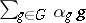,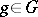,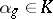, the operations being defined by the formulas: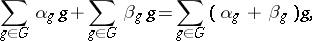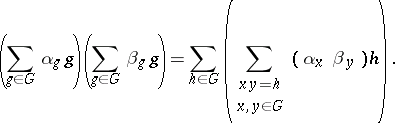(The sum on the right-hand side of the second formula is also finite.) This algebra is denoted by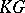; the elements ofform a basis of this algebra; multiplication of basis elements in the group algebra is induced by the group multiplication. The algebrais isomorphic to the algebra of functions defined onwith values inwhich assume only a finite number of non-zero values; in this algebra multiplication is the convolution of these functions.

The same construction can also be considered for the case whenis an associative ring. One thus arrives at the concept of the group ring of a groupover a ring; ifis commutative and has a unit element, the group ring is often called the group algebra of the group over the ring as well.

Group algebras were introduced by G. Frobenius and I. Schur  in connection with the study of group representations, since studying the representations ofover a fieldis equivalent to studying modules over the group algebra. Thus, Maschke's theorem is formulated in the language of group algebras as follows: Ifis a finite group andis a field, then the group algebrais semi-simple if and only if the order ofis not divisible by the characteristic of.

In the early 1950s group algebras of infinite groups were studied in the context of integer group algebras in algebraic topology, and for the investigation of the structure of groups. This was also promoted by a number of problems on group algebras, the best known of which is whether or not the group algebra of a torsion-free group contains zero divisors (Kaplansky's problem).

## Some directions in studies on group rings and algebras.

Radicality and semi-simplicity. A group ring has a non-zero nilpotent ideal if and only ifhas a non-zero nilpotent ideal or if the order of some finite normal subgroup inis divisible by the order of an element of the additive group of the ring. Ifis a ring without nil ideals and if the order of each element ofis not divisible by the order of any element of the additive group of, thenhas no nil ideals. The group algebraover a field of characteristic zero is semi-simple, i.e. has vanishing Jacobson radical, ifcontains a transcendental element over the field of rational numbers.

Imbedding of a group algebra into a skew-field. The group algebra of an ordered group is imbeddable in a skew-field (the Mal'tsev–von Neumann theorem). It is believed that this is also true for any right-ordered group.

Connection between ring-theoretic properties of the group ringwith the structure of the groupand the ring. As an example,is primary if and only if the ringis primary and if the grouphas no finite normal subgroups.

The isomorphism problem: If the group ringsandare isomorphic as-algebras, what is the connection between the structures of the groupsand. In particular, when areandisomorphic? It was found that a solvable torsion group of class two is uniquely determined by its group ring over the ring of integers, and that a countable Abelian-group is uniquely determined by its group ring over a ring of characteristic.

Different generalizations of the concept of a group algebra have been considered. An example is the concept of the cross product of a group and a ring, which retains many properties of a group algebra.